# Kerala Syllabus 9th Standard Physics Solutions Chapter 2 Equations of Motion

You can Download Equations of Motion Questions and Answers, Summary, Activity, Notes, Kerala Syllabus 9th Standard Physics Solutions Part 1 Chapter 2 help you to revise complete Syllabus and score more marks in your examinations.

## Kerala State Syllabus 9th Standard Physics Solutions Chapter 2 Equations of Motion

### Equations of Motion Textual Questions and Answers

Kerala Syllabus 9th Standard Physics Notes Chapter 2 Question 1.
Draw Position – Time graph. What is the nature of the grap?

 X-axis, time (s) 0 1 2 3 Y-axis, position (m) 0 1 2 3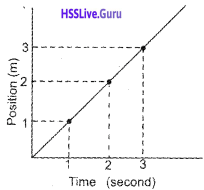The graph is straight line.

Class 9 Physics Chapter 2 Kerala Syllabus Question 2.
Using the data given below, draw a position-time graph

 Time (s) 0 1 2 3 4 5 6 Position (m) 0 2 4 6 8 10 12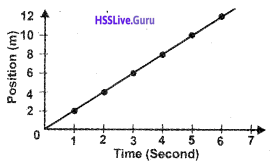Physics Class 9 Chapter 2 Equations Of Motion Kerala Syllabus Question 3.
The position-time graph regarding the motion of a car is given. Find out from the graph the distance traveled by the car in 8 s.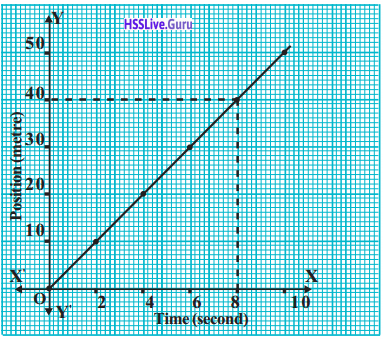Draw a perpendicular to the position-time graph at the eighth second. From the point where the perpendicular meets the graph, draw another perpendicular to the Y-axis. The point at which this perpendicular meets the Y-axis is the distance traveled by the car in 8 sec. ie 40m.
The distance covered by the car is 8s = 40 m

9th Class Physics Chapter 2 Equations Of Motion Kerala Syllabus Question 4.
Complete the table using the data from the following position-time graph related to the motion of a car.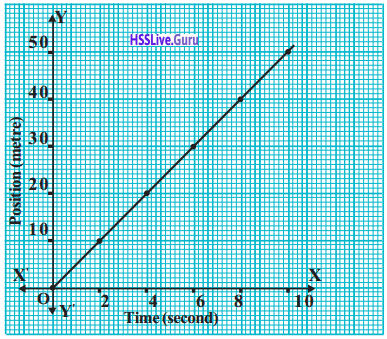Position (m) 0 2 4 6 8 10 Velocity (m/s) 0 10 20 30 40 50

Change the scale and draw another graph.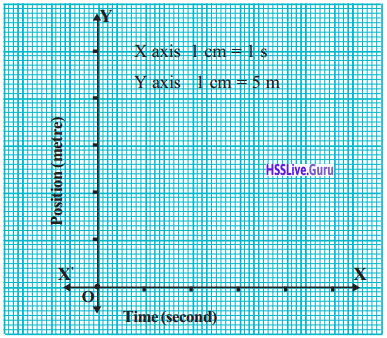Displacement of the body in 5s from both graphs is 25 m
We use scales while drawing a graph, to contain the given measurements on a graph paper. The size of the graph decreases as we increase the scale considered. But the value doesn’t change.
Motion of a car is shown below using a diagram.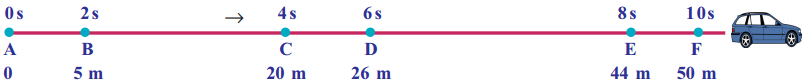Complete the table with the help of the figure

 Position (m) 0 2 4 6 8 10 Velocity (m/s) 0 5 20 36 44 50Position – time graph of a body moving with uniform speed will be a stright line. The body will be moving with nonuniform speed when the graph is not in a straight line.

9th Class Physics Notes Kerala Syllabus  Question 5.
Using the graph find out the displacement of the car in 3 s.
Displacement = 11 m

Kerala Syllabus 9th Standard Physics Notes Question 6.3
From the graph find out the time taken by the car to travel a distance of 45 m.
Time = 8.4s

Velocity – Time Graph

 Time (s) 0 2 4 6 8 10 Velocity (m/s) 10 10 10 10 10 10

With the given data in the table, draw a velocity-time graph.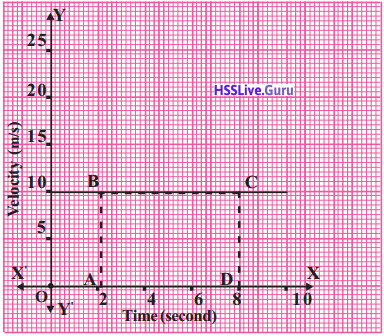The displacement of the object between the 2nd and 8th second
= Velocity × time
= AB × AD = 10 × 6 = 60 m
= Area of ABCD
= Area of the portion under the part BC of the graph
Displacement of a body within a definite interval of time is equal to the area of the portion under velocity-time graph.

Equations Of Motion

The velocity-time graph of an object traveling with uniform acceleration (freely falling stone) is given below.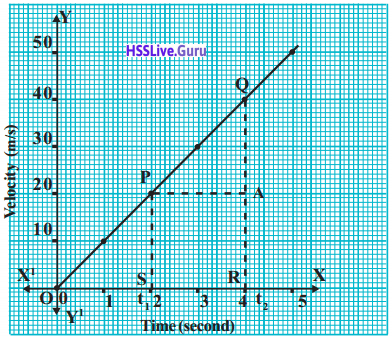PS = AR
= u
QR = v
= t2 – t1
AQ = QR – AR
= v- u
Formatin of equation showing velocity – time relation.
Acceleration = $$\frac{\text { Change in velocity }}{\text { time }}$$v- u = at
v = u + at.
This is the first equation of motion.
Formation of equation showing Position- Time Relation
Displacement = area of trapezium PQRS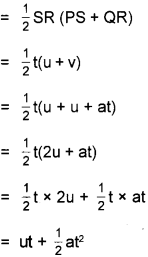S = ut + $$\frac { 1 }{ 2 }$$ at2 this is known as second equation of motion.
Formation of equation showing Position – Velocity Relation
Displacement = The area of the quadrilateralThis equation helps us to calculate the final velocity v of an object using u, a and s, even if the time taken to travel is unknown.
v2 = u2 + 2as
Equations of motion
v = u+at
s = ut + $$\frac { 1 }{ 2 }$$ at2
v2 = u2 + 2as

9th Class Physics Equations Of Motion Kerala Syllabus Question 7.
The velocity of a body starting from rest is 20 m/s in the 4th second and 40 m/s in the 8th second. What is the distance traveled by the body between the 4th and 8th second?
Velocity at the 4th second u = 20 m/s
Velocity at the 8th second v = 40 m/s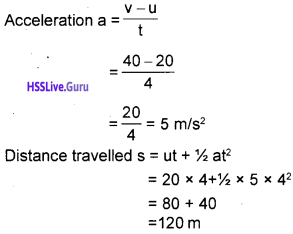9th Physics Notes Kerala Syllabus Question 8.
A car came to rest when brake was applied for 4s to get a retardation of 3 m/s2. Calculate how far the car would have traveled after applying the brake.
a = -3 m/s2
t = 4s
v = 0
v = u+at
-u = -3 × 4 + 0
u =12 m/s
Displacement of the car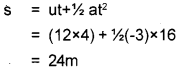Kerala Syllabus 9th Standard Physics Notes Chapter 1 Question 9.
If the velocity of a car moving with uniform velocity changes from 20 m/s to 40 m/s in 5s
a) What is the acceleration of the car?
b) What is the displacement by the car during this time interval?
a) u = 20 m/s,
v = 40 m/s,
t= 5 sKerala Syllabus 9th Standard Physics Notes English Medium Question 10.
If the velocity of a train starting from rest becomes 72 km/h in 10 minutes.
a) What is the acceleration?
b) Calculate the distance traveled by the train within this time interval
a) Here , u = 0,
v= 72 km/h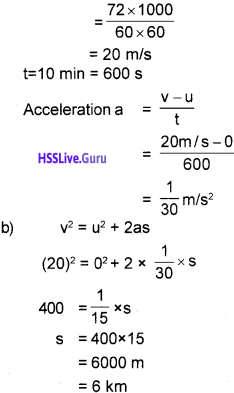Kerala Syllabus 9th Standard Physics Notes Chapter 2 Malayalam Medium Question 11.
A car starting from rest travels 100 m in 5s with uniform acceleration. Find the acceleration of the car.
u = 0,
t= 5s,
s = 100 m,
a=?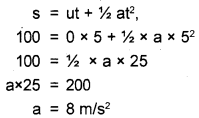Kerala Syllabus 9th Standard Physics Textbook Solutions Question 12.
An object starting from rest travels with an acceleration of 5 m/s2. What will be its velocity after 3 s?
u=0,
a= 5m/s2,
t= 3s,
v=?
v= u + at,
v= 0 + 5 × 3,
v= 15 m/s
Complete the table using the graph shown below. Compare.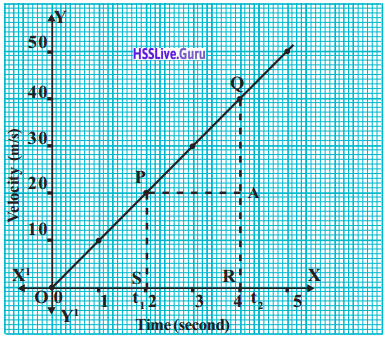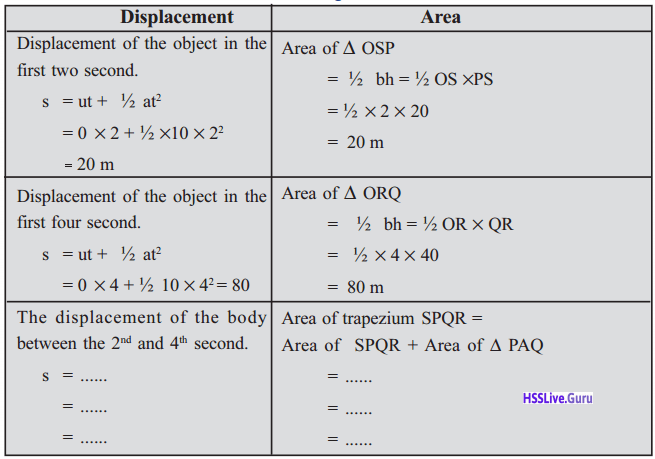In a velocity-time graph when we draw perpendiculars to the graph from the time specified, we get a geometrical shape. The area of this geometrical shape will give displacement in the specified time interval.

Let Us Assess

Question 1.
Draw position-time graph

 Time (s) 0 3 6 9 12 15 18 Position (m) 0 5 10 15 20 25 30Question 2.
Draw speed – time graph

 Time (s) 0 2 4 6 8 10 Speed (m/s) 10 15 20 20 20 15Question 3.
Examine the graph and answer the following questions.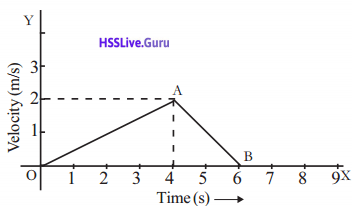a) Is the motion of the object uniform/nonuniform?
b) Say whether the acceleration from O to A is uniform? What about from A to B?
a) Non- uniform
b) O → A. uniform acceleration (0.4 m/s2)
A → B Uniform retardation (1m/s2)

Question 4.
Complete the table by analyzing the graphPosition of the object in the graph Nature of the motion From A to B Velocity increase From B to C Uniform velocity From C to D Velocity decreases

Question 5.
If a velocity of a train which starts from rest is 72 km/h (20 m/s) after 5 minutes, find out its acceleration and the distance traveled by the train in this time.Question 6.
A car attains a velocity of 54 km/h (15 m/s) within 5 seconds from an initial velocity of 18 km/h (5m/s). Calculate its acceleration and displacement.
u = 5 m/s .
t = 5s
v = 15 m/s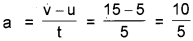= 2 m/s2
s = ut + 1/2 at2
= 5 × 5 + 1/2 × 2 × 52
= 25 + 25
= 50 m

Question 7.
Analyze the graphs given below.a) Which graph indicates uniform velocity?
b) Which graph indicates nonuniform acceleration?
c) Which graph indicates the motion of freely falling stone?
a) graph (2)
b) graph (4)
c) graph (3)

### Equations of Motion More Questions and Answers

Question 1.
Write the equations of motion. What does each letter indicate?
v = u + at
s = ut +1/2 at2
v2 = u2 + 2as
Where
u – Initial velocity
v – final velocity
a – acceleration
t – time
s – Distance (Displacement)

Question 2.
An object starting form rest travels with a uniform acceleration of 5 m/s2. Calculate the velocity and distance traveled after 1 minute?
v = u + at
u = 0
= 0+ 5m/s2 x 60 s
a = 5m/s2
= 0+ 300 m/s
t = 1
mt = 60 sec
= 300 m/s
s =ut + 1/2 at2
= 0 × 60 + 1/2 × 5 × 602
= 9000 m

Question 3.
Draw a velocity-time graph on the basis of the given table.

 Time (s) 0 5 10 15 20 25 30 35 40 Velocity(m/s) 0 10 20 20 20 15 10 5 0